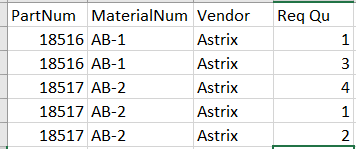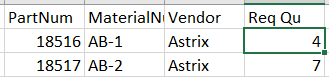# DataTable has same data but quantity column values are different, Sum the quantity column and make into single row

Hi All,

I have a query regarding datatable, In my scenario, I have data like PartNum, MaterialNum, and quantity, I want to merge into a single row when PartNum and MaterialNum are the same but the quantity should be the sum of all the rows, Please see the sample data below. Kindly help me with this.

Input:Required Output:Hi,

Could you use pivot table for this excel table so that you would get desired ouput with accumulating (sum of) your quantity values.

@ramesh_kola
we can doit with a group by and sum up the group members

Assign activity:
LHS: dtResult | dataType: DataTable
RHS: YourOriginDataTableVar.Clone

Assign Activity:
LHS: dtResult
RHS:

``````(From d in YourOriginDataTableVar.AsEnumerable
Group d by k1=d("PartNum").toString.Trim, k2=d("MaterialNum").toString.Trim into grp=Group
Let s = grp.Sum(Function (x) CInt(x("Req Qu").toString.Trim))
Let ra = new Object(){k1,k2,grp.First()("Vendor"),s}